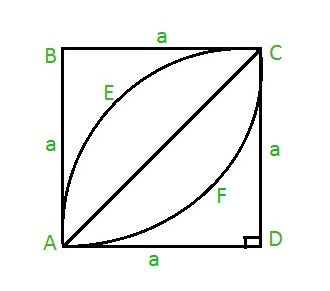# Area of a leaf inside a square

Given an integer a as the side of the square ABCD. The task is to find the area of the leaf AECFA inside the square as shown below:Examples:

Input: a = 7
Output: 28

Input: a = 21
Output: 252

## Recommended: Please try your approach on {IDE} first, before moving on to the solution.

Approach: To calculate the area of the leaf, first find the area of the half leaf AECA, which can be given as:

Area of half leaf = Area of quadrant AECDA – Area of right triangle ACD.
Thus, Area of half leaf = ( PI * a * a / 4 ) – a * a / 2 where PI = 22 / 7 and a is the side of the square.
Hence, the area of full leaf will be ( PI * a * a / 2 ) – a * a
On taking a * a common we get, Area of leaf = a * a ( PI / 2 – 1 )

Below is the implementation of the above approach:

## C

 `// C program to find the area of ` `// leaf inside a square ` `#include ` `#define PI 3.14159265 ` ` `  `// Function to find area of ` `// leaf ` `float` `area_leaf(``float` `a) ` `{ ` `    ``return` `(a * a * (PI / 2 - 1)); ` `} ` ` `  `// Driver code ` `int` `main() ` `{ ` `    ``float` `a = 7; ` `    ``printf``(``"%f"``, ` `           ``area_leaf(a)); ` `    ``return` `0; ` `} `

## Java

 `// Java code to find the area of ` `// leaf inside a square ` `import` `java.lang.*; ` ` `  `class` `GFG { ` ` `  `    ``static` `double` `PI = ``3.14159265``; ` ` `  `    ``// Function to find the area of ` `    ``// leaf ` `    ``public` `static` `double` `area_leaf(``double` `a) ` `    ``{ ` `        ``return` `(a * a * (PI / ``2` `- ``1``)); ` `    ``} ` ` `  `    ``// Driver code ` `    ``public` `static` `void` `main(String[] args) ` `    ``{ ` `        ``double` `a = ``7``; ` `        ``System.out.println(area_leaf(a)); ` `    ``} ` `} `

## Python3

 `# Python3 code to find the area of leaf  ` `# inside a square ` `PI ``=` `3.14159265` `     `  `# Function to find the area of  ` `# leaf ` `def` `area_leaf( a ): ` `    ``return` `( a ``*` `a ``*` `( PI ``/` `2` `-` `1` `) ) ` `     `  `# Driver code ` `a ``=` `7` `print``(area_leaf( a )) `

## C#

 `// C# code to find the area of ` `// leaf ` `// inside square ` `using` `System; ` ` `  `class` `GFG { ` `    ``static` `double` `PI = 3.14159265; ` ` `  `    ``// Function to find the area of ` `    ``// leaf ` `    ``public` `static` `double` `area_leaf(``double` `a) ` `    ``{ ` `        ``return` `(a * a * (PI / 2 - 1)); ` `    ``} ` ` `  `    ``// Driver code ` `    ``public` `static` `void` `Main() ` `    ``{ ` `        ``double` `a = 7; ` `        ``Console.Write(area_leaf(a)); ` `    ``} ` `} `

## PHP

 ` `

Output:

```28
```

My Personal Notes arrow_drop_upCheck out this Author's contributed articles.

If you like GeeksforGeeks and would like to contribute, you can also write an article using contribute.geeksforgeeks.org or mail your article to contribute@geeksforgeeks.org. See your article appearing on the GeeksforGeeks main page and help other Geeks.

Please Improve this article if you find anything incorrect by clicking on the "Improve Article" button below.